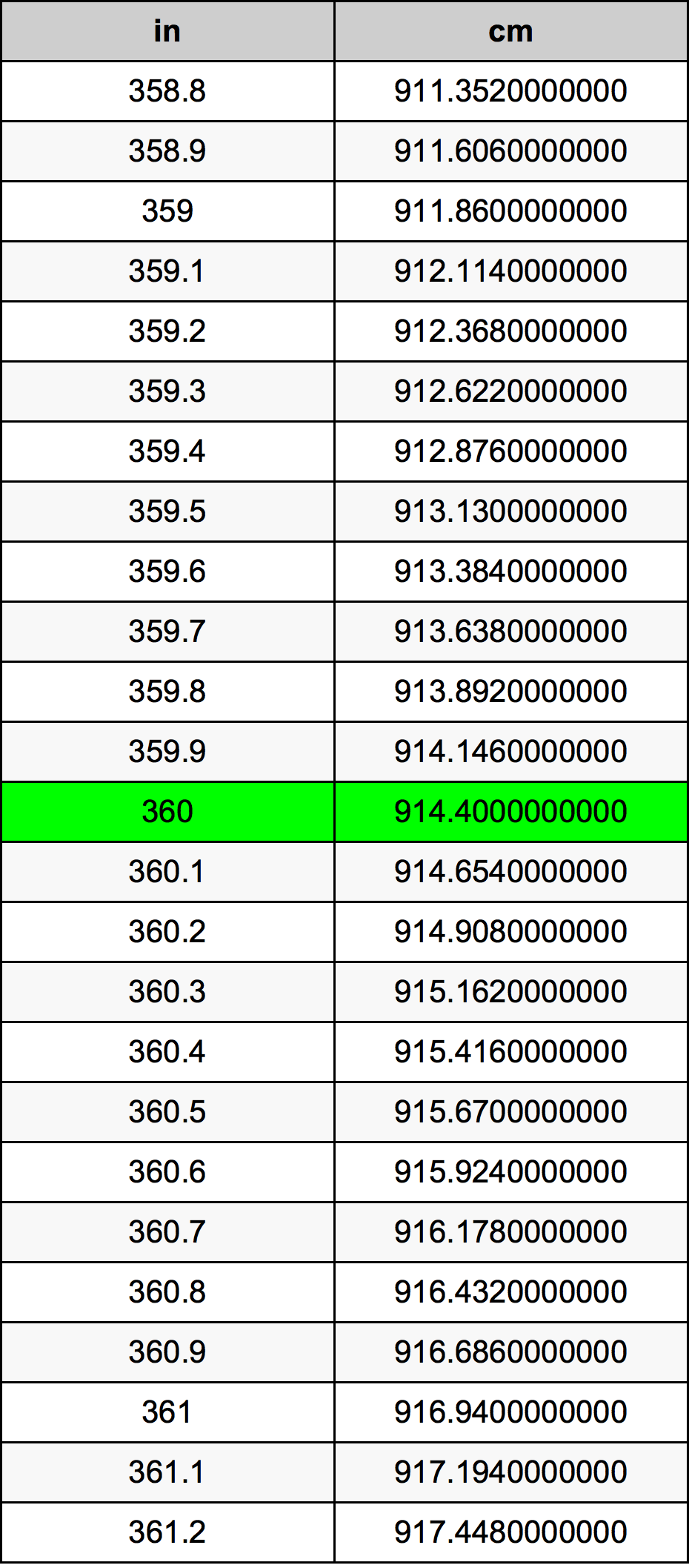Inches To Centimeters

# 360 in to cm360 Inches to Centimeters

in
=
cm

## How to convert 360 inches to centimeters?

 360 in * 2.54 cm = 914.4 cm 1 in
A common question is How many inch in 360 centimeter? And the answer is 141.732283465 in in 360 cm. Likewise the question how many centimeter in 360 inch has the answer of 914.4 cm in 360 in.

## How much are 360 inches in centimeters?

360 inches equal 914.4 centimeters (360in = 914.4cm). Converting 360 in to cm is easy. Simply use our calculator above, or apply the formula to change the length 360 in to cm.

## Convert 360 in to common lengths

UnitLengths
Nanometer9144000000.0 nm
Micrometer9144000.0 µm
Millimeter9144.0 mm
Centimeter914.4 cm
Inch360.0 in
Foot30.0 ft
Yard10.0 yd
Meter9.144 m
Kilometer0.009144 km
Mile0.0056818182 mi
Nautical mile0.004937365 nmi

## What is 360 inches in cm?

To convert 360 in to cm multiply the length in inches by 2.54. The 360 in in cm formula is [cm] = 360 * 2.54. Thus, for 360 inches in centimeter we get 914.4 cm.

## 360 Inch Conversion Table## Alternative spelling

360 Inches to Centimeters, 360 Inches in Centimeters, 360 in to Centimeters, 360 in in Centimeters, 360 Inch to cm, 360 Inch in cm, 360 in to Centimeter, 360 in in Centimeter, 360 Inch to Centimeters, 360 Inch in Centimeters, 360 Inches to cm, 360 Inches in cm, 360 Inch to Centimeter, 360 Inch in Centimeter